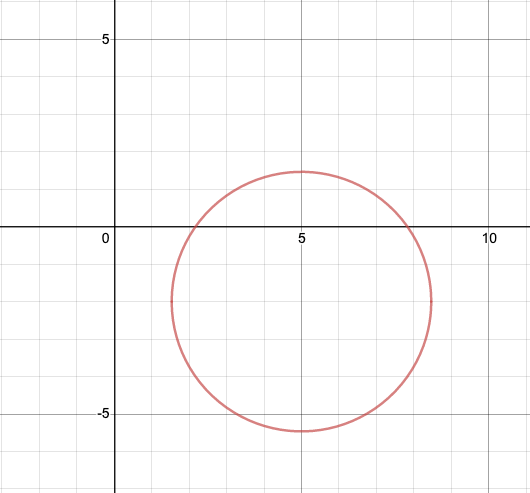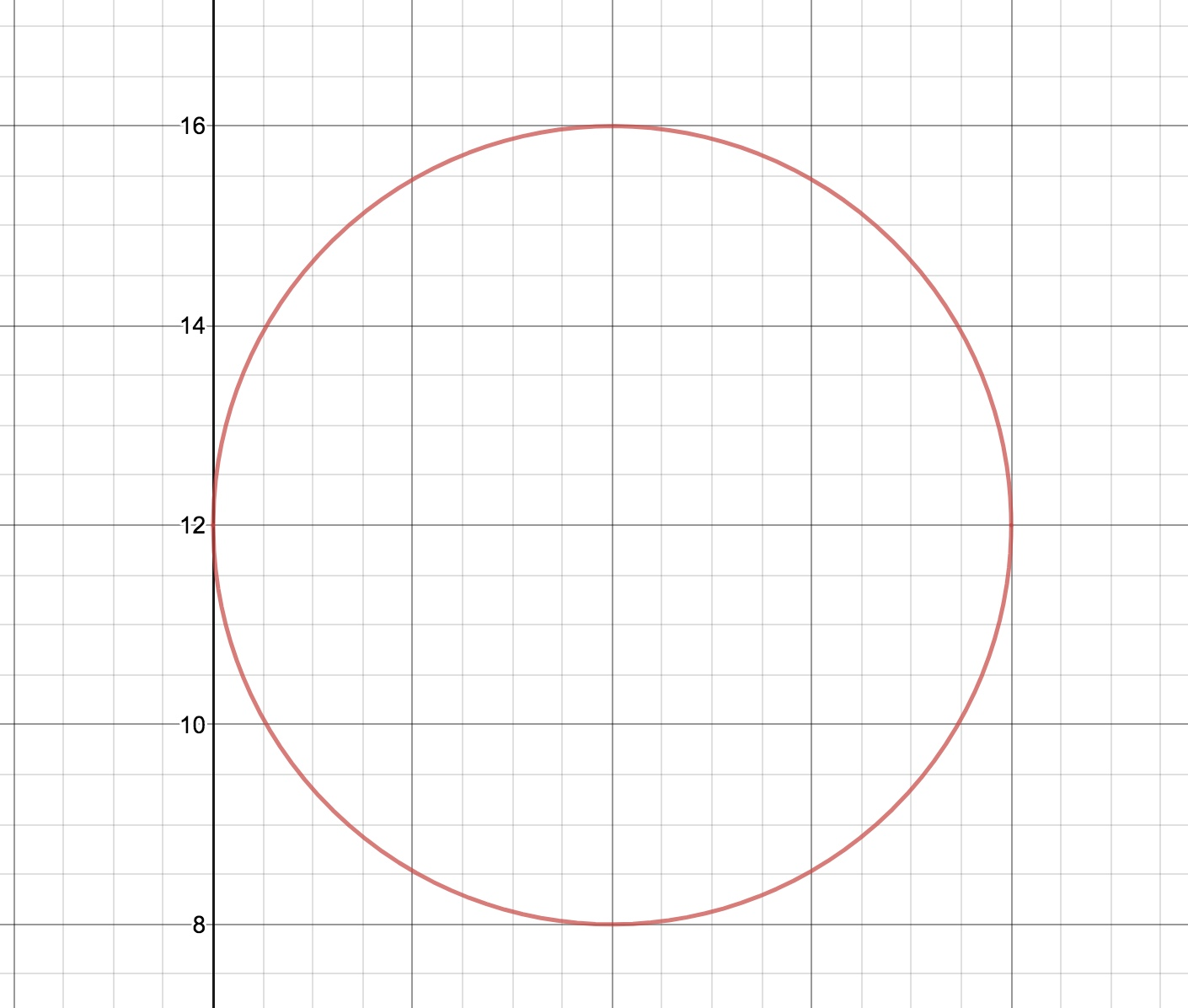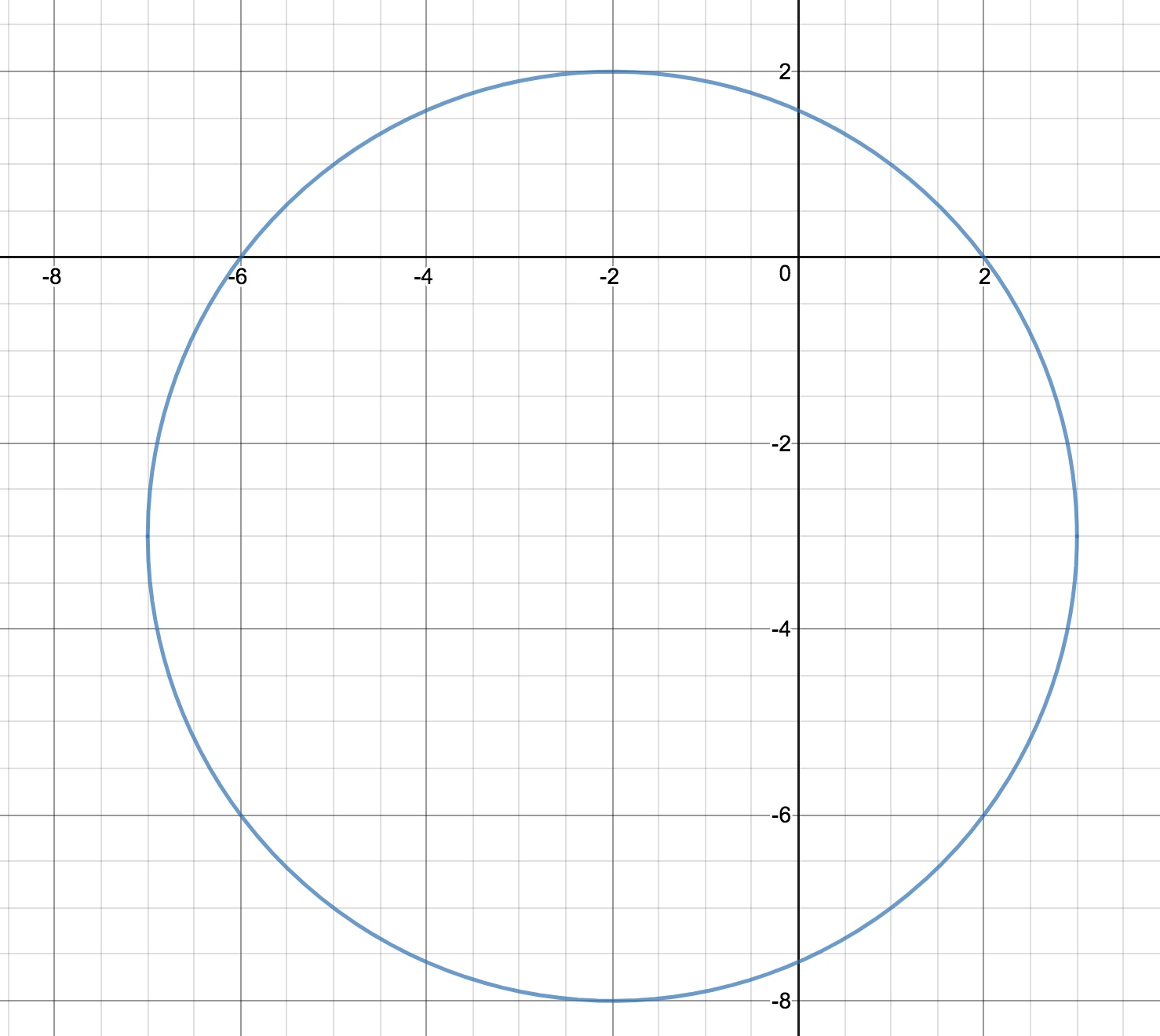# POLQ 3 | Lesson 4 | Practice (Writing in Center-Radius Form) Solutions

Solutions:

1) $$(x-5)^2+(y+2)^2 = 12$$

Center is at $$(5, -2)$$ and the radius is $$2\sqrt{3}$$2) $$(x-4)^2+(y-12)^2 = 16$$

Center at $$(4, 12)$$ and radius is 43) $$(x+2)^2+(y+3)^2 = 25$$

Center is at $$(-2, -3)$$ and the radius is 5It should be much easier to graph a circle using center-radius form since those two pieces of information are all that are needed to draw a circle..

Graphs are created using Desmos.com.